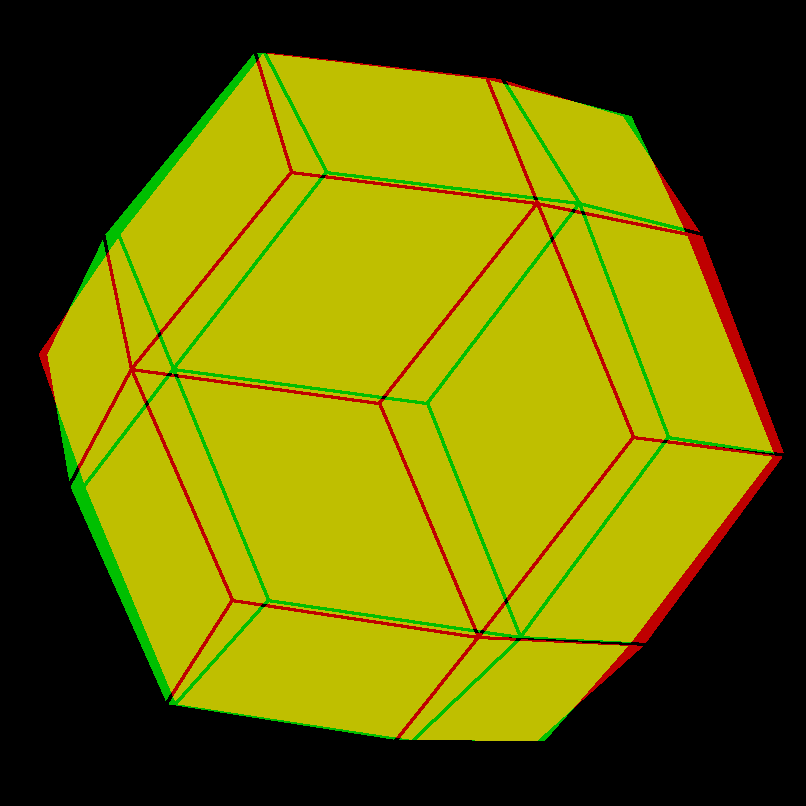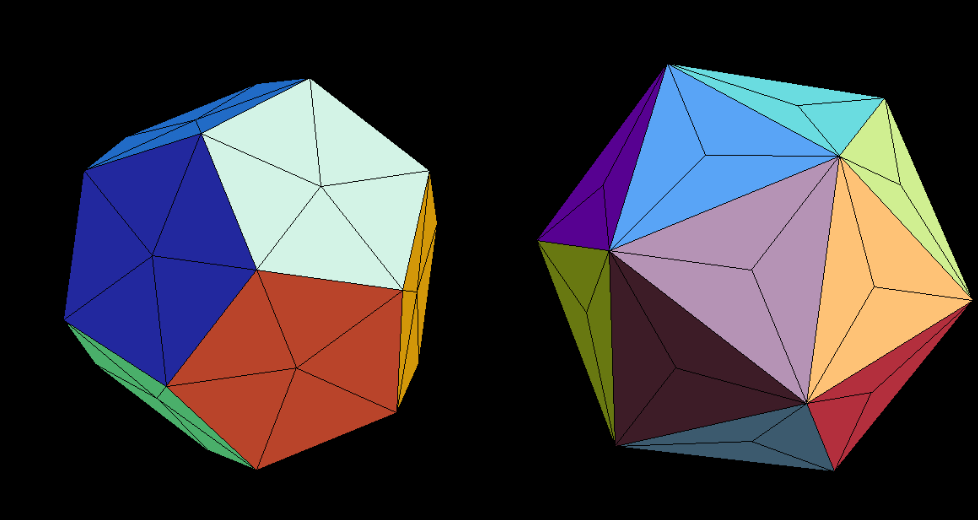# Rhombic Triacontahedron

### Dual of the IcosiDodecahedronThe Rhombic Triacontahedron can be obtained from the dodecahedron by raising pentagon pyramids on its pentagon faces and it can be obtained from the icosahedron by raising triangular pyramids on its triangular faces. The resulting triacontahedra have the same size if the icosahedron edges are by the golden ratio (sqrt(5)+1)/2 longer than the dodecahedron edge lengths. In the animation, face colors depend on the face normals; therefore one can see that in the last step neighbouring pyramid faces become coplanar, thus creating the rhombic faces of the triacontahedron with diagonal length ratio (sqrt(5)+1)/2.